Скачать презентацию Which program is better Why A define prime

• Количество слайдов: 42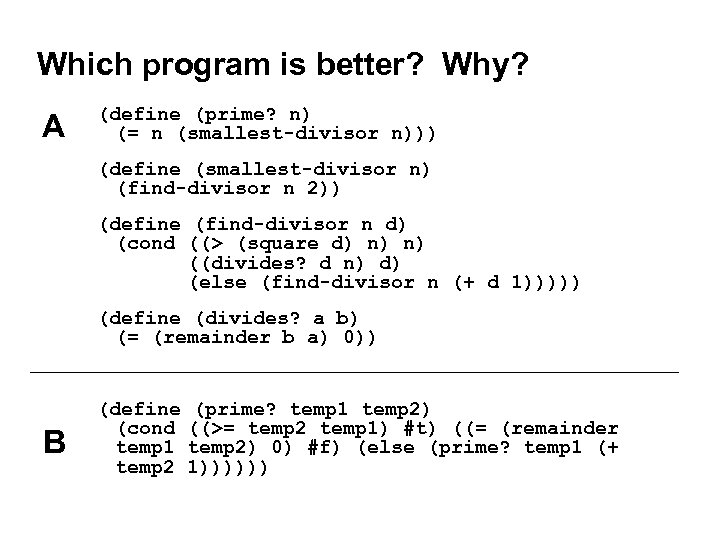Which program is better? Why? A (define (prime? n) (= n (smallest-divisor n))) (define (smallest-divisor n) (find-divisor n 2)) (define (find-divisor n d) (cond ((> (square d) n) n) ((divides? d n) d) (else (find-divisor n (+ d 1))))) (define (divides? a b) (= (remainder b a) 0)) B (define (prime? temp 1 temp 2) (cond ((>= temp 2 temp 1) #t) ((= (remainder temp 1 temp 2) 0) #f) (else (prime? temp 1 (+ temp 2 1))))))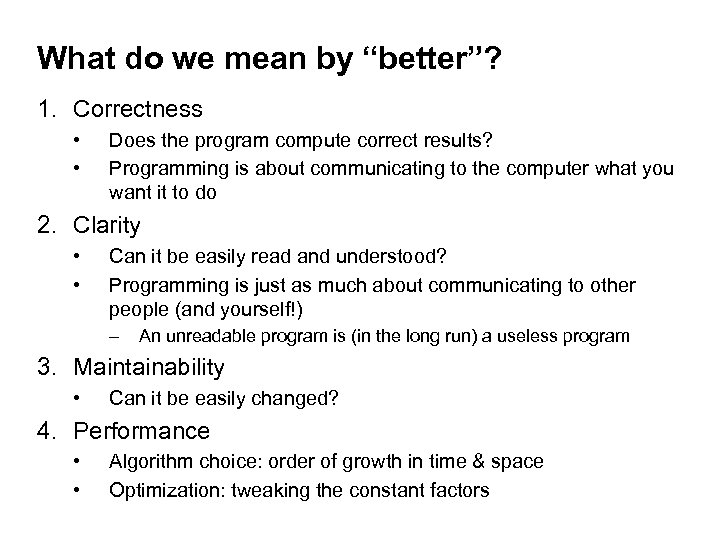What do we mean by “better”? 1. Correctness • • Does the program compute correct results? Programming is about communicating to the computer what you want it to do 2. Clarity • • Can it be easily read and understood? Programming is just as much about communicating to other people (and yourself!) – An unreadable program is (in the long run) a useless program 3. Maintainability • Can it be easily changed? 4. Performance • • Algorithm choice: order of growth in time & space Optimization: tweaking the constant factors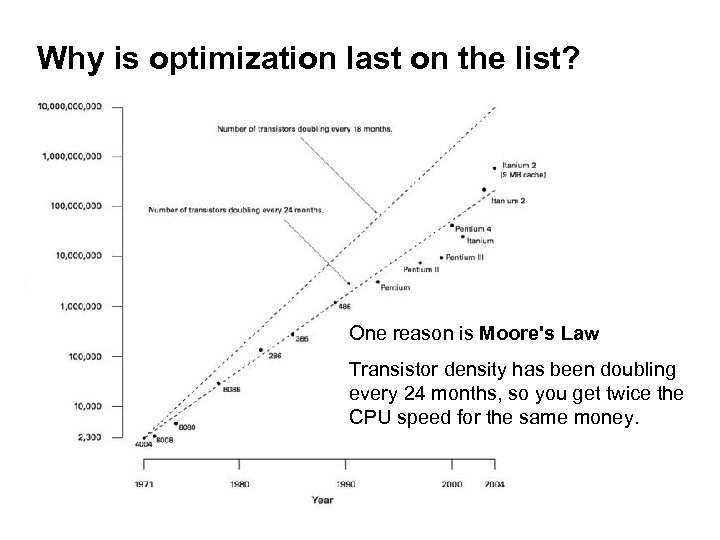Why is optimization last on the list? One reason is Moore's Law Transistor density has been doubling every 24 months, so you get twice the CPU speed for the same money.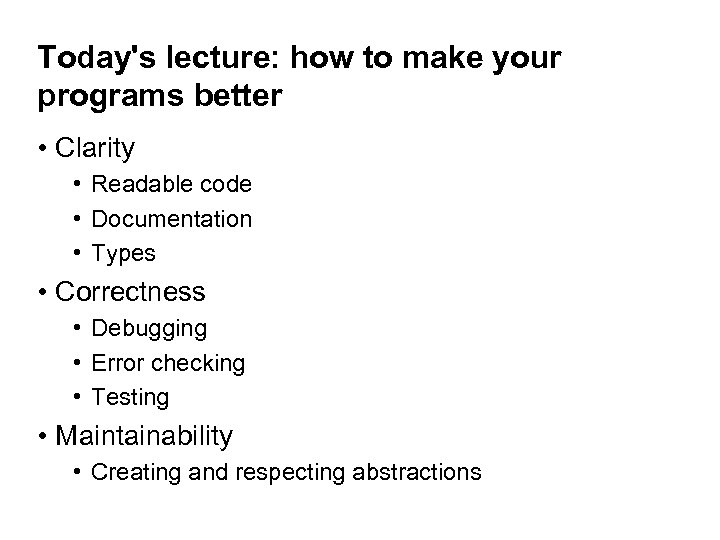Today's lecture: how to make your programs better • Clarity • Readable code • Documentation • Types • Correctness • Debugging • Error checking • Testing • Maintainability • Creating and respecting abstractions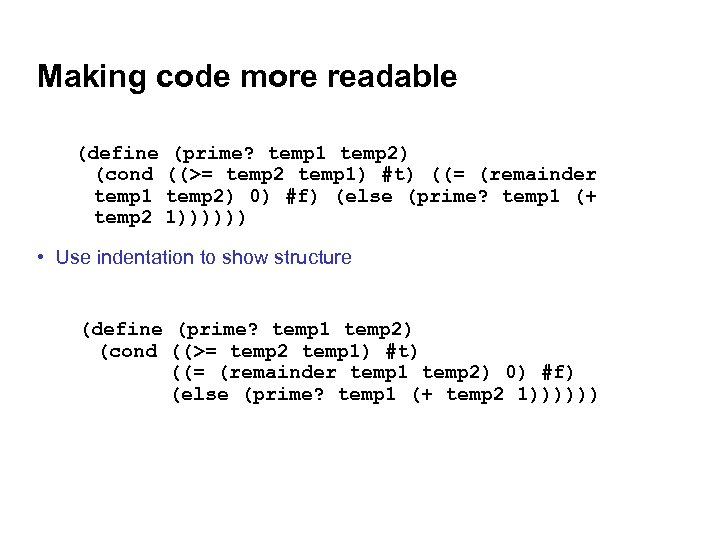Making code more readable (define (prime? temp 1 temp 2) (cond ((>= temp 2 temp 1) #t) ((= (remainder temp 1 temp 2) 0) #f) (else (prime? temp 1 (+ temp 2 1)))))) • Use indentation to show structure (define (prime? temp 1 temp 2) (cond ((>= temp 2 temp 1) #t) ((= (remainder temp 1 temp 2) 0) #f) (else (prime? temp 1 (+ temp 2 1))))))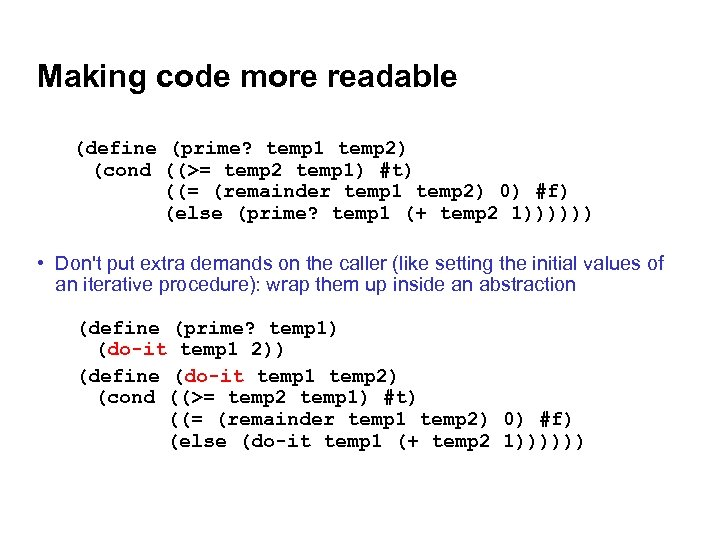Making code more readable (define (prime? temp 1 temp 2) (cond ((>= temp 2 temp 1) #t) ((= (remainder temp 1 temp 2) 0) #f) (else (prime? temp 1 (+ temp 2 1)))))) • Don't put extra demands on the caller (like setting the initial values of an iterative procedure): wrap them up inside an abstraction (define (prime? temp 1) (do-it temp 1 2)) (define (do-it temp 1 temp 2) (cond ((>= temp 2 temp 1) #t) ((= (remainder temp 1 temp 2) 0) #f) (else (do-it temp 1 (+ temp 2 1))))))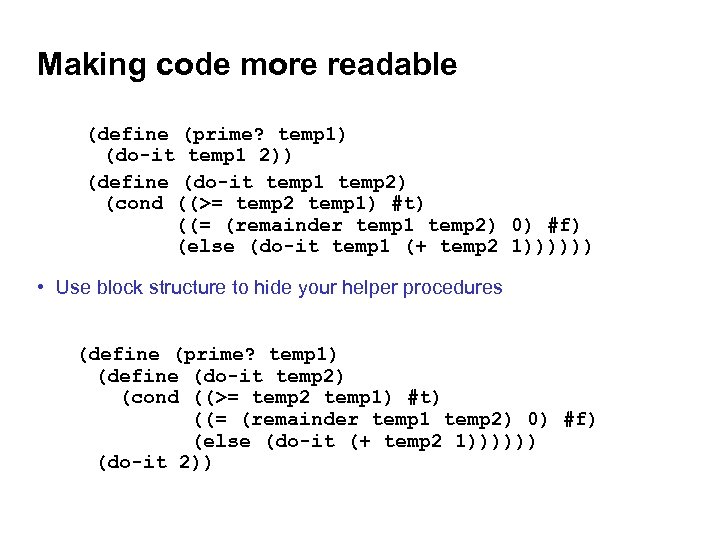Making code more readable (define (prime? temp 1) (do-it temp 1 2)) (define (do-it temp 1 temp 2) (cond ((>= temp 2 temp 1) #t) ((= (remainder temp 1 temp 2) 0) #f) (else (do-it temp 1 (+ temp 2 1)))))) • Use block structure to hide your helper procedures (define (prime? temp 1) (define (do-it temp 2) (cond ((>= temp 2 temp 1) #t) ((= (remainder temp 1 temp 2) 0) #f) (else (do-it (+ temp 2 1)))))) (do-it 2))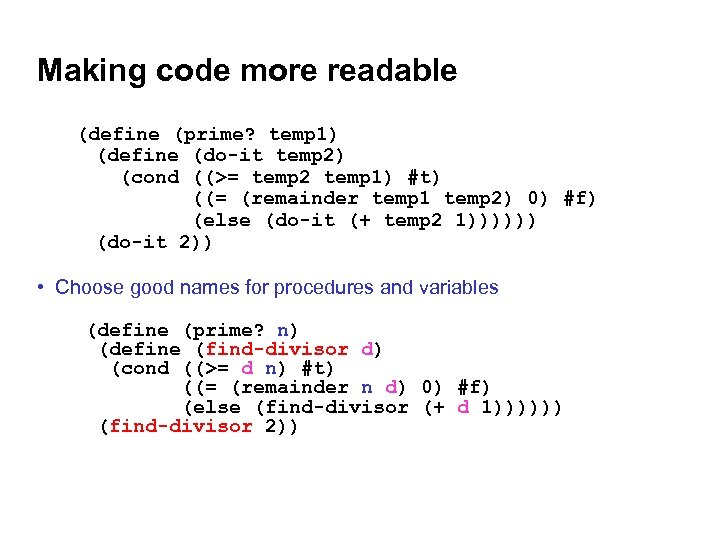Making code more readable (define (prime? temp 1) (define (do-it temp 2) (cond ((>= temp 2 temp 1) #t) ((= (remainder temp 1 temp 2) 0) #f) (else (do-it (+ temp 2 1)))))) (do-it 2)) • Choose good names for procedures and variables (define (prime? n) (define (find-divisor d) (cond ((>= d n) #t) ((= (remainder n d) 0) #f) (else (find-divisor (+ d 1)))))) (find-divisor 2))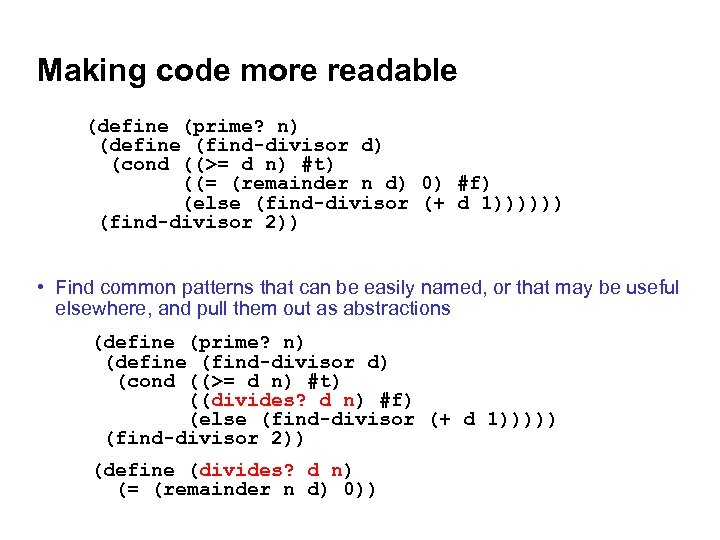Making code more readable (define (prime? n) (define (find-divisor d) (cond ((>= d n) #t) ((= (remainder n d) 0) #f) (else (find-divisor (+ d 1)))))) (find-divisor 2)) • Find common patterns that can be easily named, or that may be useful elsewhere, and pull them out as abstractions (define (prime? n) (define (find-divisor d) (cond ((>= d n) #t) ((divides? d n) #f) (else (find-divisor (+ d 1))))) (find-divisor 2)) (define (divides? d n) (= (remainder n d) 0))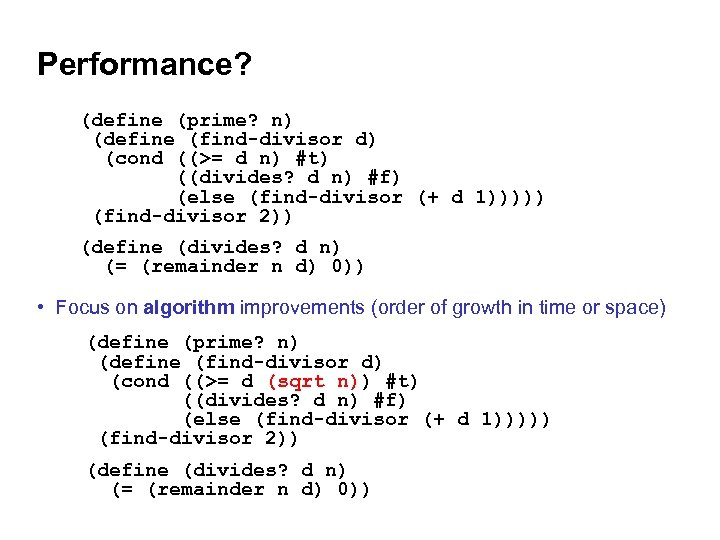Performance? (define (prime? n) (define (find-divisor d) (cond ((>= d n) #t) ((divides? d n) #f) (else (find-divisor (+ d 1))))) (find-divisor 2)) (define (divides? d n) (= (remainder n d) 0)) • Focus on algorithm improvements (order of growth in time or space) (define (prime? n) (define (find-divisor d) (cond ((>= d (sqrt n)) #t) ((divides? d n) #f) (else (find-divisor (+ d 1))))) (find-divisor 2)) (define (divides? d n) (= (remainder n d) 0))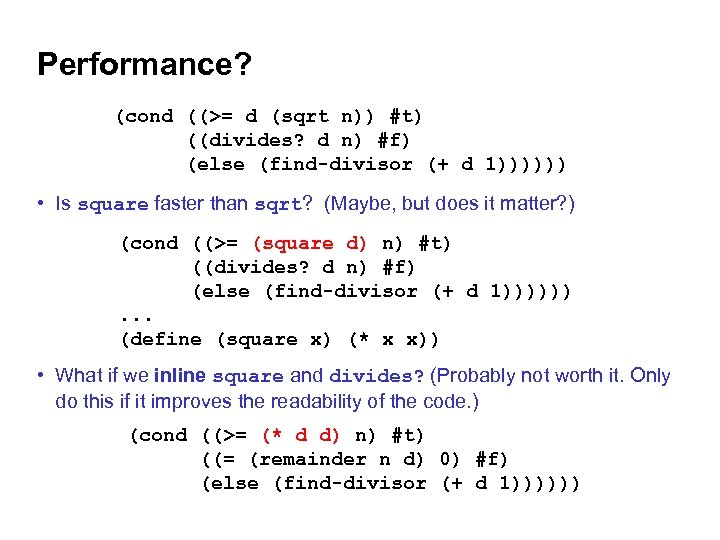Performance? (cond ((>= d (sqrt n)) #t) ((divides? d n) #f) (else (find-divisor (+ d 1)))))) • Is square faster than sqrt? (Maybe, but does it matter? ) (cond ((>= (square d) n) #t) ((divides? d n) #f) (else (find-divisor (+ d 1)))))). . . (define (square x) (* x x)) • What if we inline square and divides? (Probably not worth it. Only do this if it improves the readability of the code. ) (cond ((>= (* d d) n) #t) ((= (remainder n d) 0) #f) (else (find-divisor (+ d 1))))))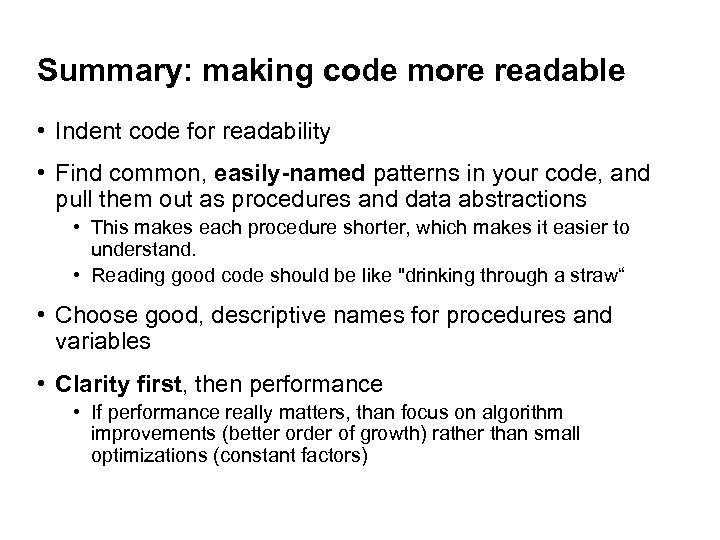Summary: making code more readable • Indent code for readability • Find common, easily-named patterns in your code, and pull them out as procedures and data abstractions • This makes each procedure shorter, which makes it easier to understand. • Reading good code should be like "drinking through a straw“ • Choose good, descriptive names for procedures and variables • Clarity first, then performance • If performance really matters, than focus on algorithm improvements (better order of growth) rather than small optimizations (constant factors)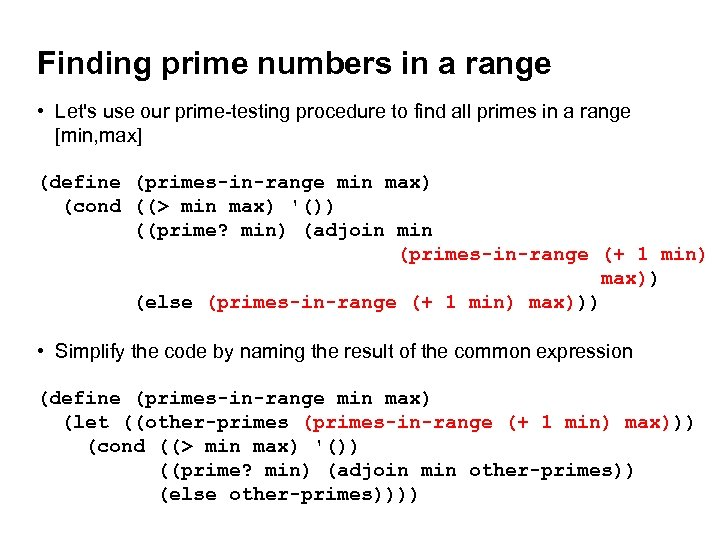Finding prime numbers in a range • Let's use our prime-testing procedure to find all primes in a range [min, max] (define (primes-in-range min max) (cond ((> min max) '()) ((prime? min) (adjoin min (primes-in-range (+ 1 min) max)) (else (primes-in-range (+ 1 min) max))) • Simplify the code by naming the result of the common expression (define (primes-in-range min max) (let ((other-primes (primes-in-range (+ 1 min) max))) (cond ((> min max) '()) ((prime? min) (adjoin min other-primes)) (else other-primes))))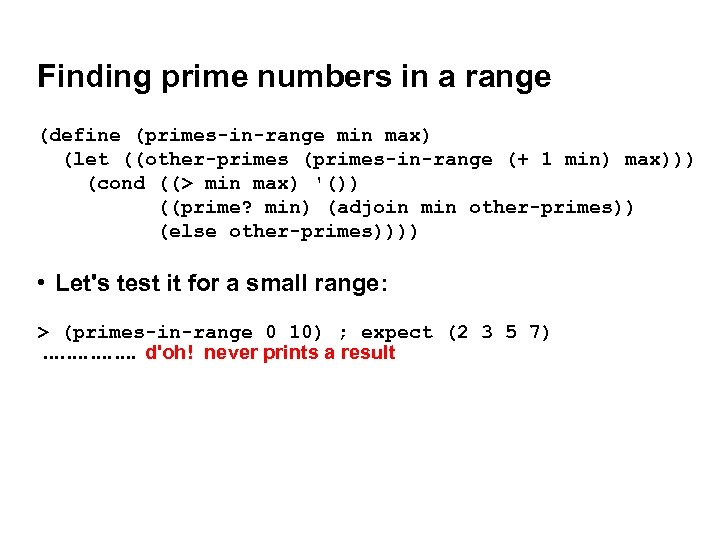Finding prime numbers in a range (define (primes-in-range min max) (let ((other-primes (primes-in-range (+ 1 min) max))) (cond ((> min max) '()) ((prime? min) (adjoin min other-primes)) (else other-primes)))) • Let's test it for a small range: > (primes-in-range 0 10) ; expect (2 3 5 7). . . . d'oh! never prints a result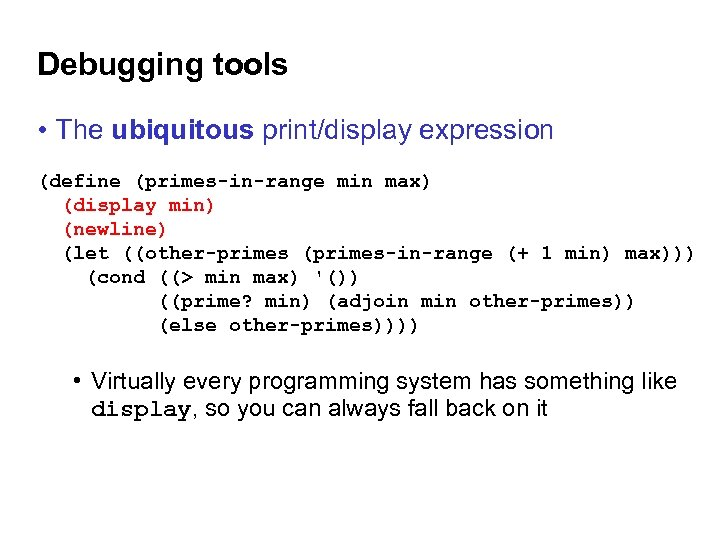Debugging tools • The ubiquitous print/display expression (define (primes-in-range min max) (display min) (newline) (let ((other-primes (primes-in-range (+ 1 min) max))) (cond ((> min max) '()) ((prime? min) (adjoin min other-primes)) (else other-primes)))) • Virtually every programming system has something like display, so you can always fall back on it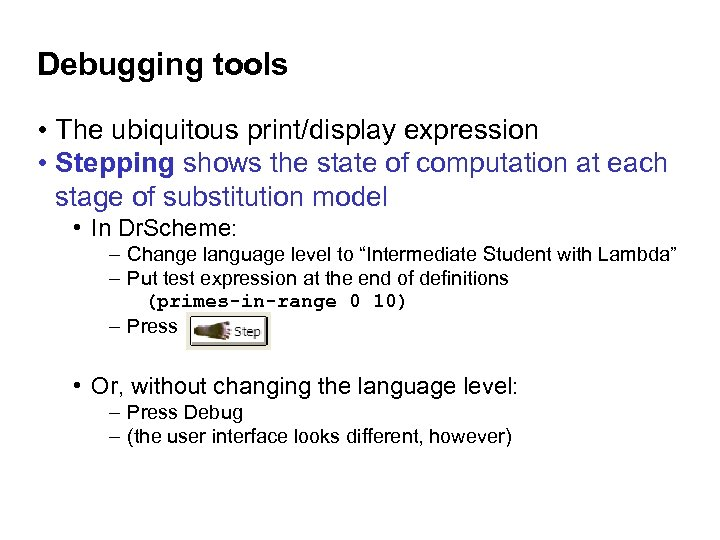Debugging tools • The ubiquitous print/display expression • Stepping shows the state of computation at each stage of substitution model • In Dr. Scheme: – Change language level to “Intermediate Student with Lambda” – Put test expression at the end of definitions (primes-in-range 0 10) – Press • Or, without changing the language level: – Press Debug – (the user interface looks different, however)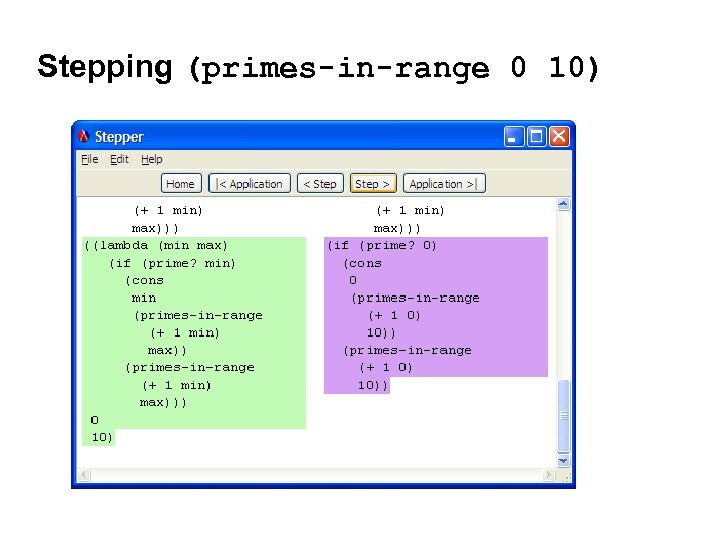Stepping (primes-in-range 0 10)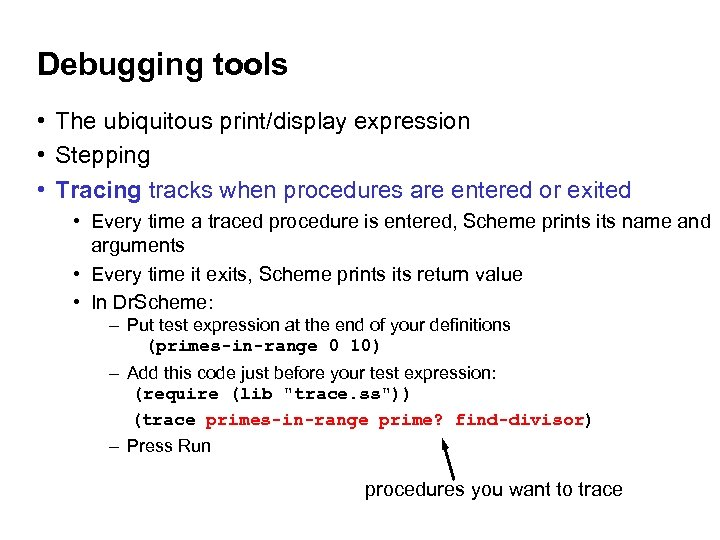Debugging tools • The ubiquitous print/display expression • Stepping • Tracing tracks when procedures are entered or exited • Every time a traced procedure is entered, Scheme prints its name and arguments • Every time it exits, Scheme prints its return value • In Dr. Scheme: – Put test expression at the end of your definitions (primes-in-range 0 10) – Add this code just before your test expression: (require (lib "trace. ss")) (trace primes-in-range prime? find-divisor) – Press Run procedures you want to trace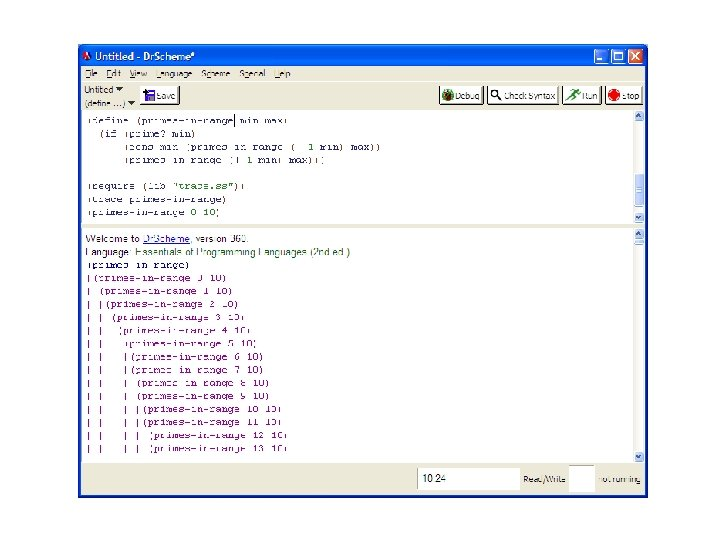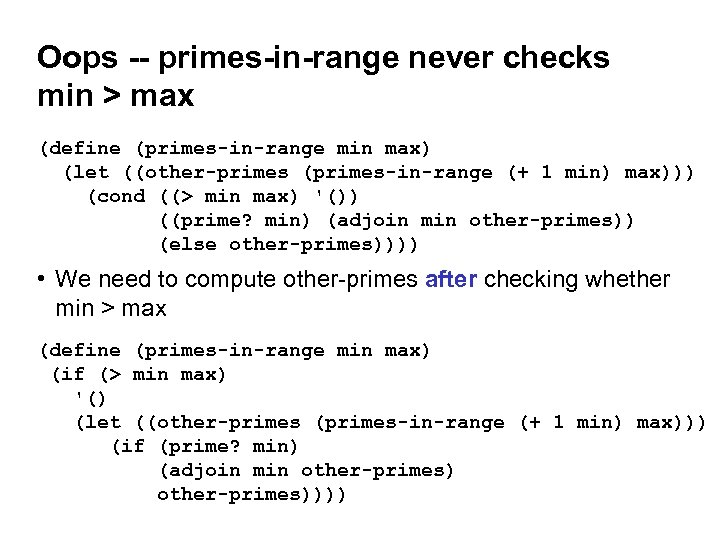Oops -- primes-in-range never checks min > max (define (primes-in-range min max) (let ((other-primes (primes-in-range (+ 1 min) max))) (cond ((> min max) '()) ((prime? min) (adjoin min other-primes)) (else other-primes)))) • We need to compute other-primes after checking whether min > max (define (primes-in-range min max) (if (> min max) '() (let ((other-primes (primes-in-range (+ 1 min) max))) (if (prime? min) (adjoin min other-primes))))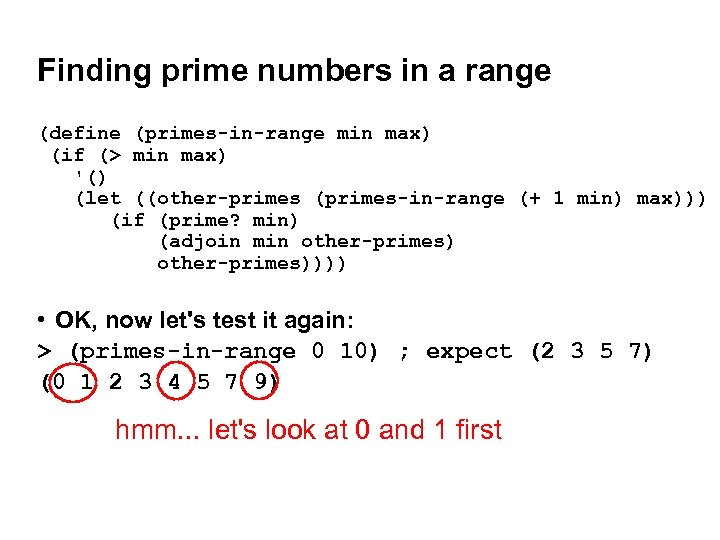Finding prime numbers in a range (define (primes-in-range min max) (if (> min max) '() (let ((other-primes (primes-in-range (+ 1 min) max))) (if (prime? min) (adjoin min other-primes)))) • OK, now let's test it again: > (primes-in-range 0 10) ; expect (2 3 5 7) (0 1 2 3 4 5 7 9) hmm. . . let's look at 0 and 1 first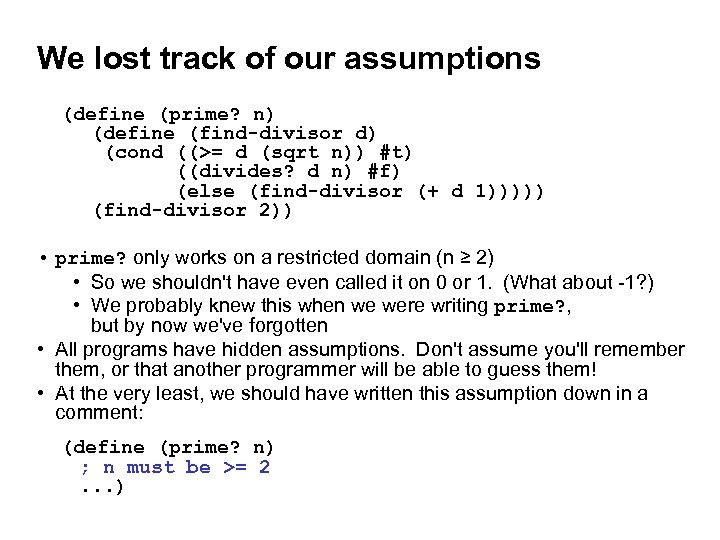We lost track of our assumptions (define (prime? n) (define (find-divisor d) (cond ((>= d (sqrt n)) #t) ((divides? d n) #f) (else (find-divisor (+ d 1))))) (find-divisor 2)) • prime? only works on a restricted domain (n ≥ 2) • So we shouldn't have even called it on 0 or 1. (What about -1? ) • We probably knew this when we were writing prime? , but by now we've forgotten • All programs have hidden assumptions. Don't assume you'll remember them, or that another programmer will be able to guess them! • At the very least, we should have written this assumption down in a comment: (define (prime? n) ; n must be >= 2 . . . )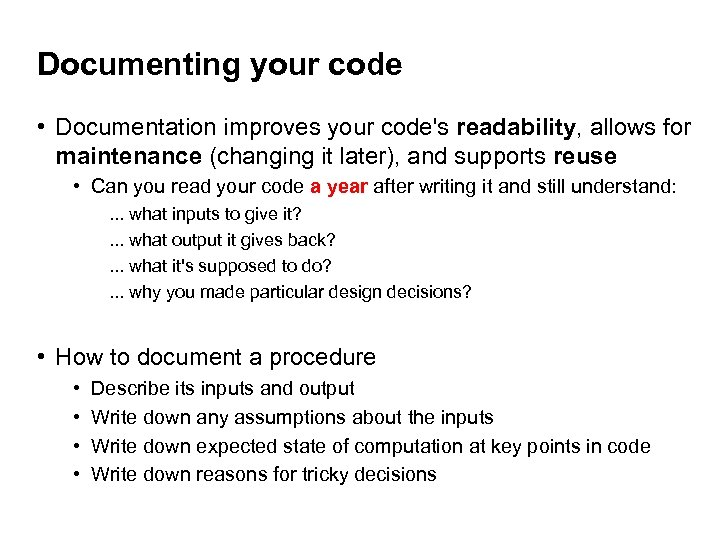Documenting your code • Documentation improves your code's readability, allows for maintenance (changing it later), and supports reuse • Can you read your code a year after writing it and still understand: . . . what inputs to give it? . . . what output it gives back? . . . what it's supposed to do? . . . why you made particular design decisions? • How to document a procedure • • Describe its inputs and output Write down any assumptions about the inputs Write down expected state of computation at key points in code Write down reasons for tricky decisions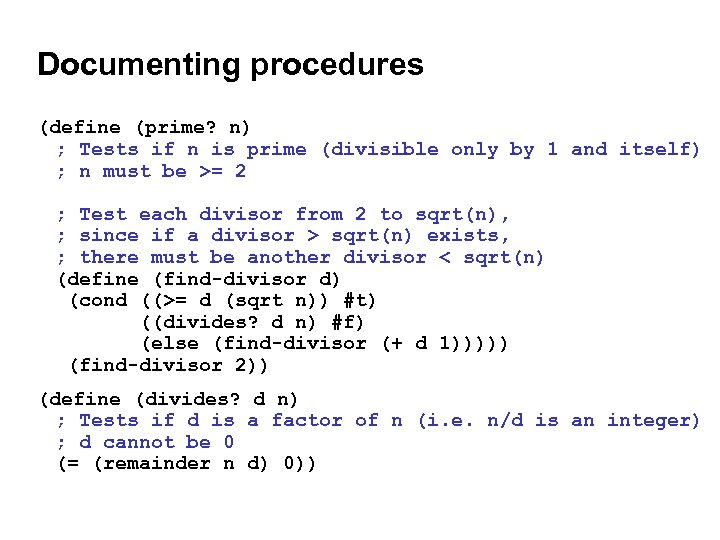Documenting procedures (define (prime? n) ; Tests if n is prime (divisible only by 1 and itself) ; n must be >= 2 ; Test each divisor from 2 to sqrt(n), ; since if a divisor > sqrt(n) exists, ; there must be another divisor < sqrt(n) (define (find-divisor d) (cond ((>= d (sqrt n)) #t) ((divides? d n) #f) (else (find-divisor (+ d 1))))) (find-divisor 2)) (define (divides? d n) ; Tests if d is a factor of n (i. e. n/d is an integer) ; d cannot be 0 (= (remainder n d) 0))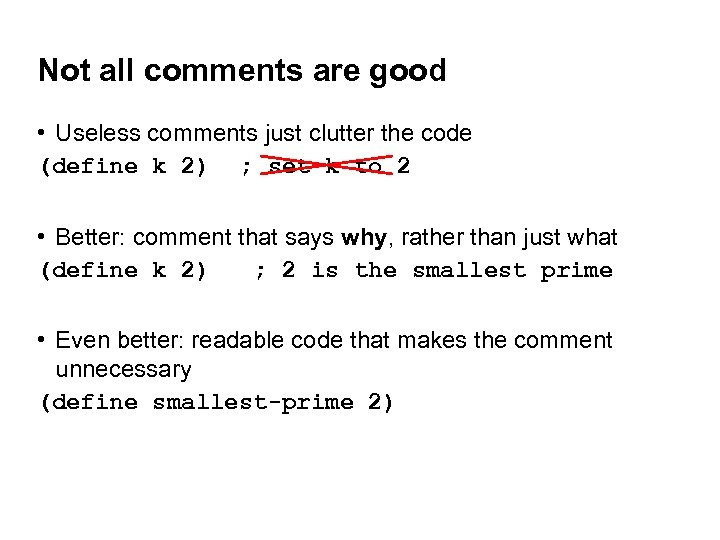Not all comments are good • Useless comments just clutter the code (define k 2) ; set k to 2 • Better: comment that says why, rather than just what (define k 2) ; 2 is the smallest prime • Even better: readable code that makes the comment unnecessary (define smallest-prime 2)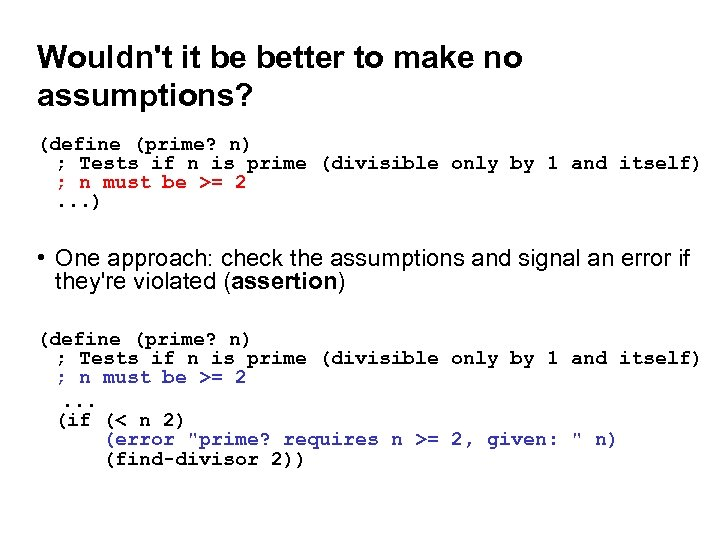Wouldn't it be better to make no assumptions? (define (prime? n) ; Tests if n is prime (divisible only by 1 and itself) ; n must be >= 2 . . . ) • One approach: check the assumptions and signal an error if they're violated (assertion) (define (prime? n) ; Tests if n is prime (divisible only by 1 and itself) ; n must be >= 2 . . . (if (< n 2) (error "prime? requires n >= 2, given: " n) (find-divisor 2))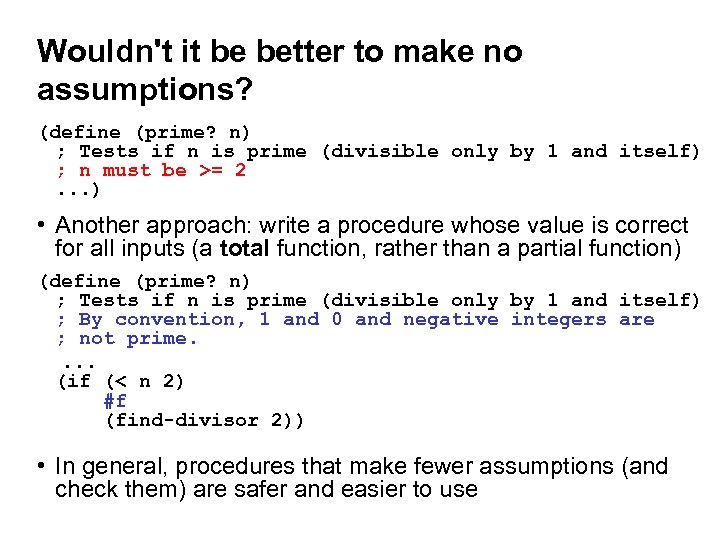Wouldn't it be better to make no assumptions? (define (prime? n) ; Tests if n is prime (divisible only by 1 and itself) ; n must be >= 2 . . . ) • Another approach: write a procedure whose value is correct for all inputs (a total function, rather than a partial function) (define (prime? n) ; Tests if n is prime (divisible only by 1 and itself) ; By convention, 1 and 0 and negative integers are ; not prime. . . . (if (< n 2) #f (find-divisor 2)) • In general, procedures that make fewer assumptions (and check them) are safer and easier to use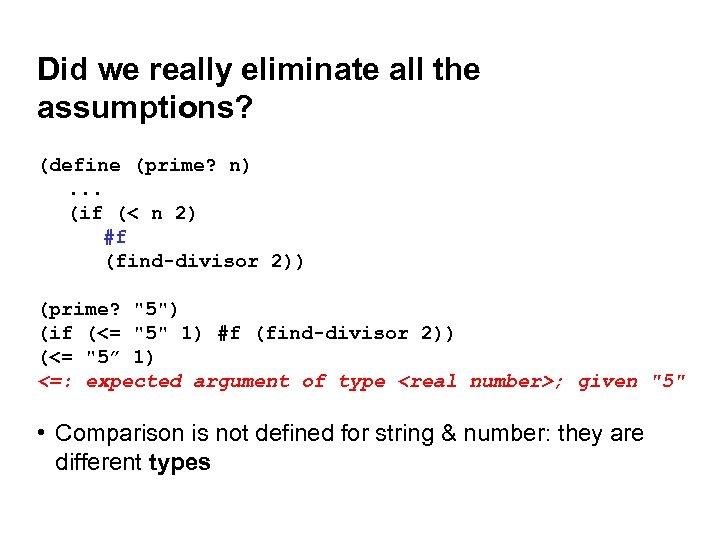Did we really eliminate all the assumptions? (define (prime? n) . . . (if (< n 2) #f (find-divisor 2)) (prime? "5") (if (<= "5" 1) #f (find-divisor 2)) (<= "5” 1) <=: expected argument of type ; given "5" • Comparison is not defined for string & number: they are different types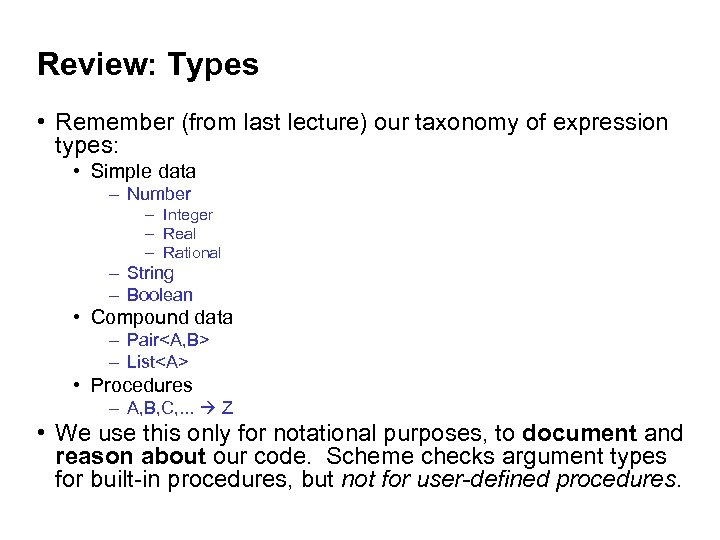Review: Types • Remember (from last lecture) our taxonomy of expression types: • Simple data – Number – Integer – Real – Rational – String – Boolean • Compound data – Pair – List • Procedures – A, B, C, . . . Z • We use this only for notational purposes, to document and reason about our code. Scheme checks argument types for built-in procedures, but not for user-defined procedures.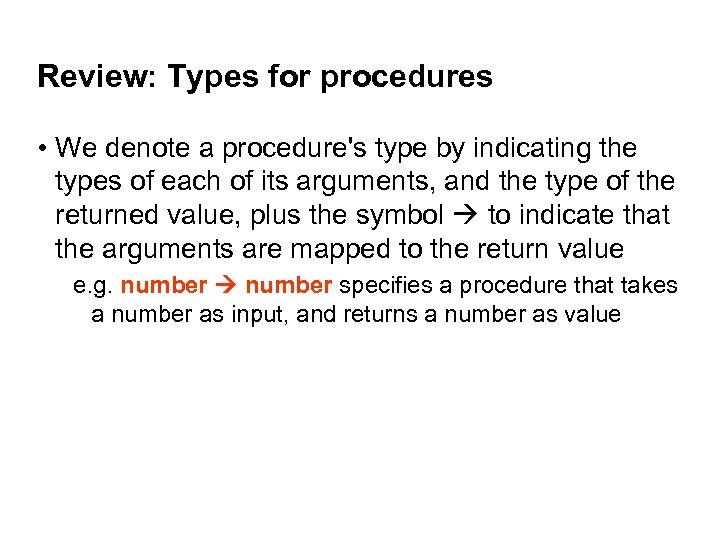Review: Types for procedures • We denote a procedure's type by indicating the types of each of its arguments, and the type of the returned value, plus the symbol to indicate that the arguments are mapped to the return value e. g. number specifies a procedure that takes a number as input, and returns a number as value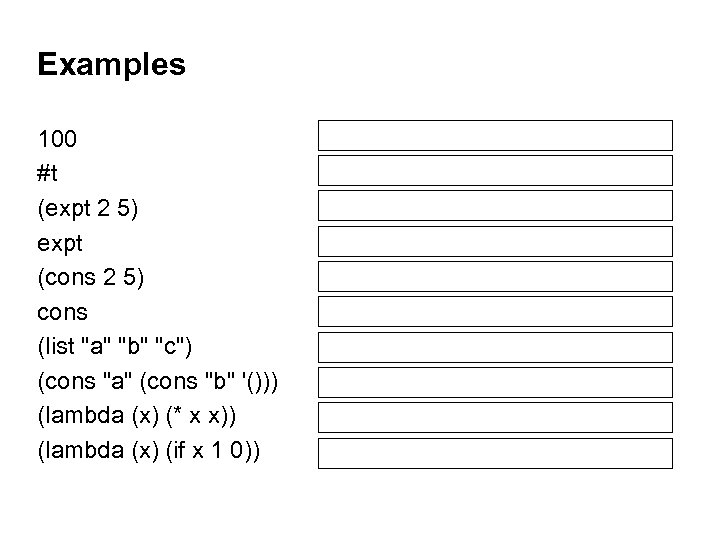Examples 100 #t (expt 2 5) expt (cons 2 5) cons (list "a" "b" "c") (cons "a" (cons "b" '())) (lambda (x) (* x x)) (lambda (x) (if x 1 0)) ; number ; boolean ; number, number ; pair ; A, B pair ; list ; number ; boolean number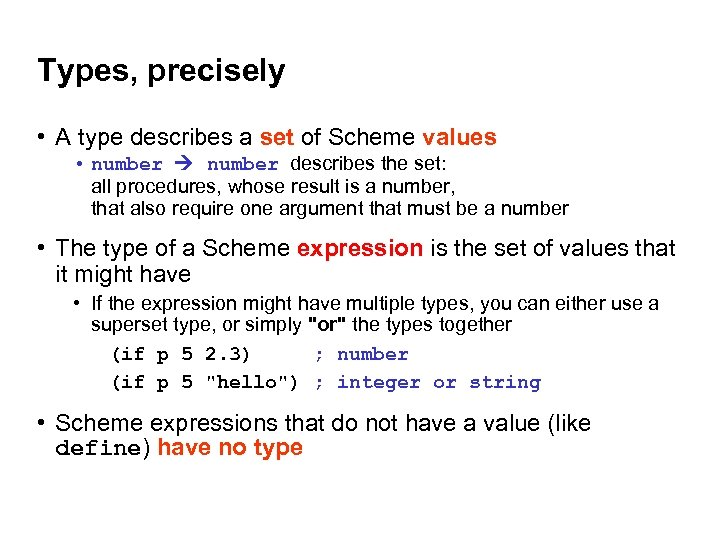Types, precisely • A type describes a set of Scheme values • number describes the set: all procedures, whose result is a number, that also require one argument that must be a number • The type of a Scheme expression is the set of values that it might have • If the expression might have multiple types, you can either use a superset type, or simply "or" the types together (if p 5 2. 3) ; number (if p 5 "hello") ; integer or string • Scheme expressions that do not have a value (like define) have no type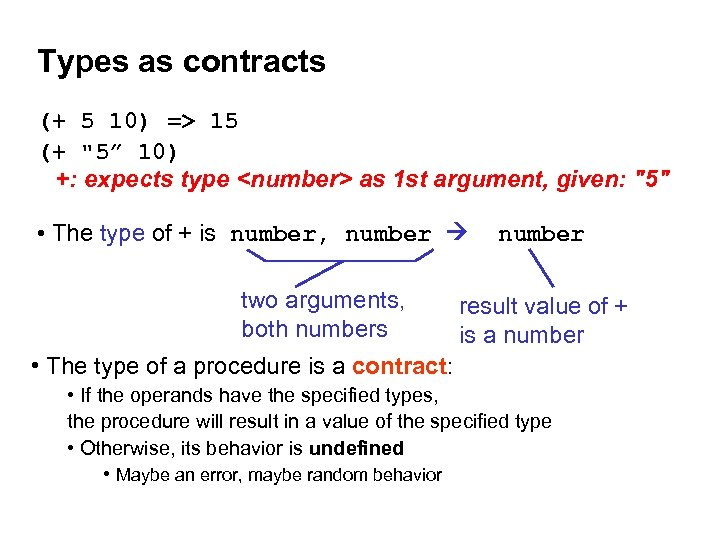Types as contracts (+ 5 10) => 15 (+ "5” 10) +: expects type as 1 st argument, given: "5" • The type of + is number, number two arguments, both numbers result value of + is a number • The type of a procedure is a contract: • If the operands have the specified types, the procedure will result in a value of the specified type • Otherwise, its behavior is undefined • Maybe an error, maybe random behavior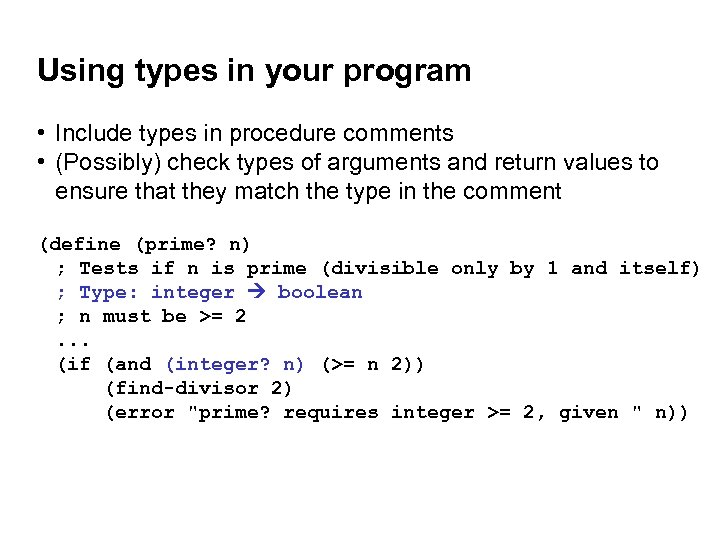Using types in your program • Include types in procedure comments • (Possibly) check types of arguments and return values to ensure that they match the type in the comment (define (prime? n) ; Tests if n is prime (divisible only by 1 and itself) ; Type: integer boolean ; n must be >= 2. . . (if (and (integer? n) (>= n 2)) (find-divisor 2) (error "prime? requires integer >= 2, given " n))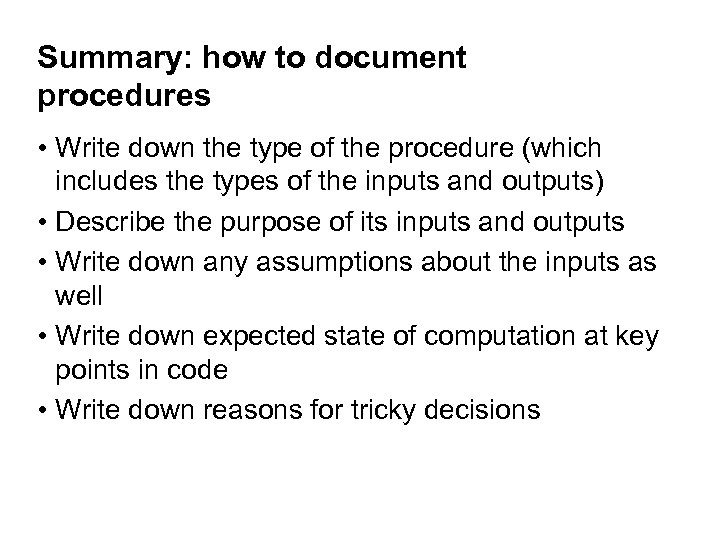Summary: how to document procedures • Write down the type of the procedure (which includes the types of the inputs and outputs) • Describe the purpose of its inputs and outputs • Write down any assumptions about the inputs as well • Write down expected state of computation at key points in code • Write down reasons for tricky decisions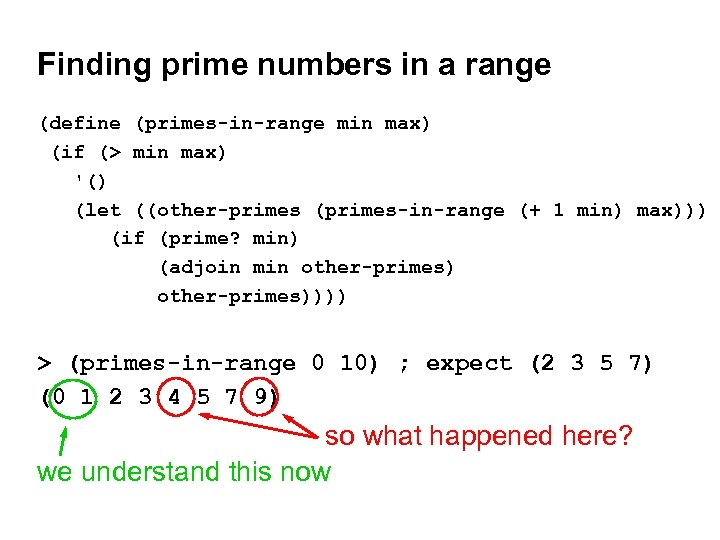Finding prime numbers in a range (define (primes-in-range min max) (if (> min max) '() (let ((other-primes (primes-in-range (+ 1 min) max))) (if (prime? min) (adjoin min other-primes)))) > (primes-in-range 0 10) ; expect (2 3 5 7) (0 1 2 3 4 5 7 9) so what happened here? we understand this now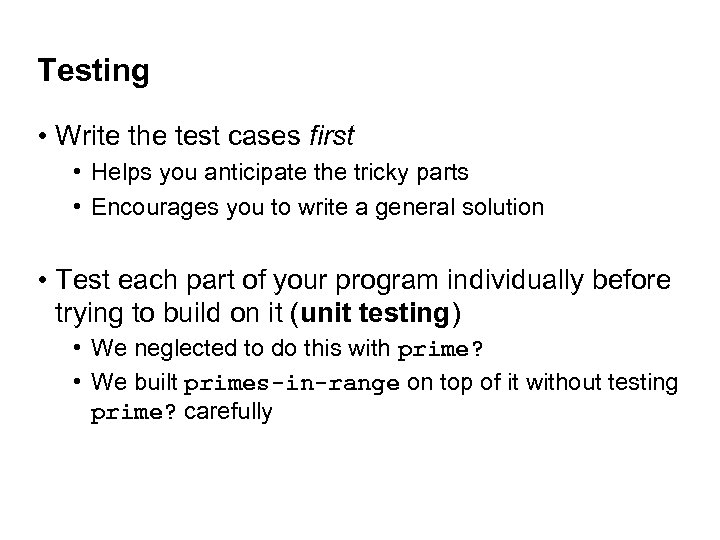Testing • Write the test cases first • Helps you anticipate the tricky parts • Encourages you to write a general solution • Test each part of your program individually before trying to build on it (unit testing) • We neglected to do this with prime? • We built primes-in-range on top of it without testing prime? carefully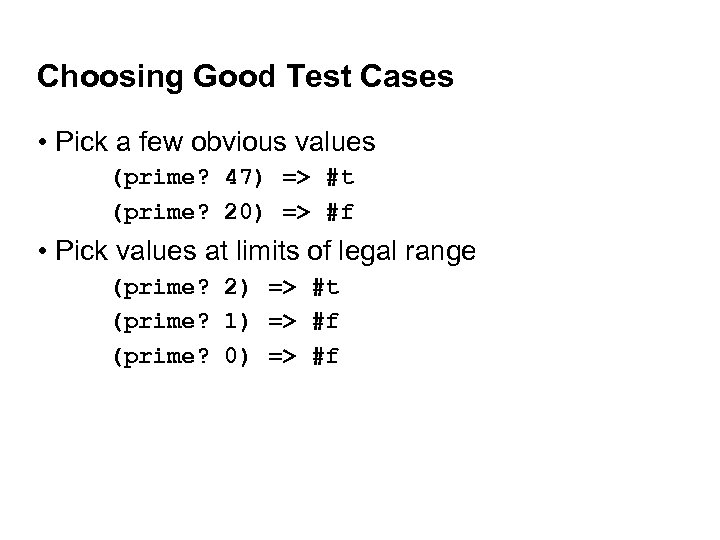Choosing Good Test Cases • Pick a few obvious values (prime? 47) => #t (prime? 20) => #f • Pick values at limits of legal range (prime? 2) => #t (prime? 1) => #f (prime? 0) => #f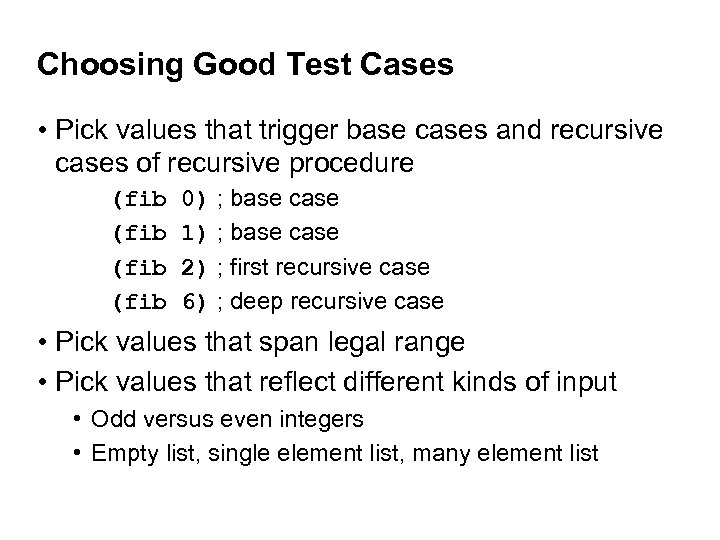Choosing Good Test Cases • Pick values that trigger base cases and recursive cases of recursive procedure (fib 0) ; base case (fib 1) ; base case (fib 2) ; first recursive case (fib 6) ; deep recursive case • Pick values that span legal range • Pick values that reflect different kinds of input • Odd versus even integers • Empty list, single element list, many element list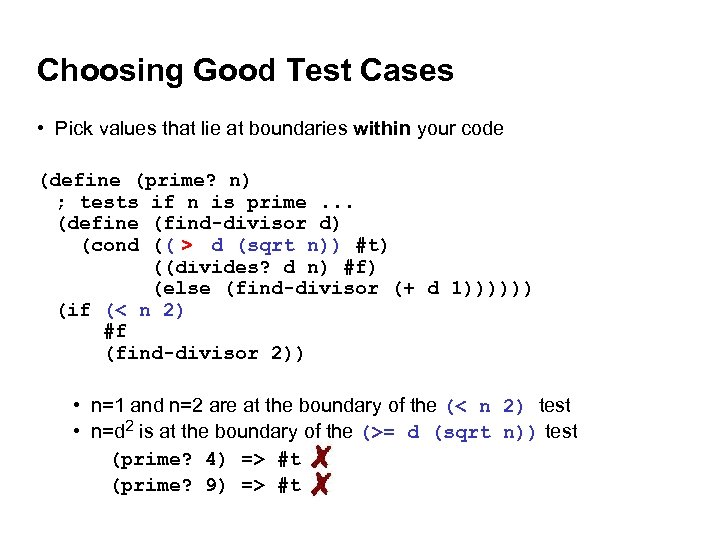Choosing Good Test Cases • Pick values that lie at boundaries within your code (define (prime? n) ; tests if n is prime. . . (define (find-divisor d) (cond ((>= d (sqrt n)) #t) > ((divides? d n) #f) (else (find-divisor (+ d 1)))))) (if (< n 2) #f (find-divisor 2)) • n=1 and n=2 are at the boundary of the (< n 2) test • n=d 2 is at the boundary of the (>= d (sqrt n)) test (prime? 4) => #t (prime? 9) => #t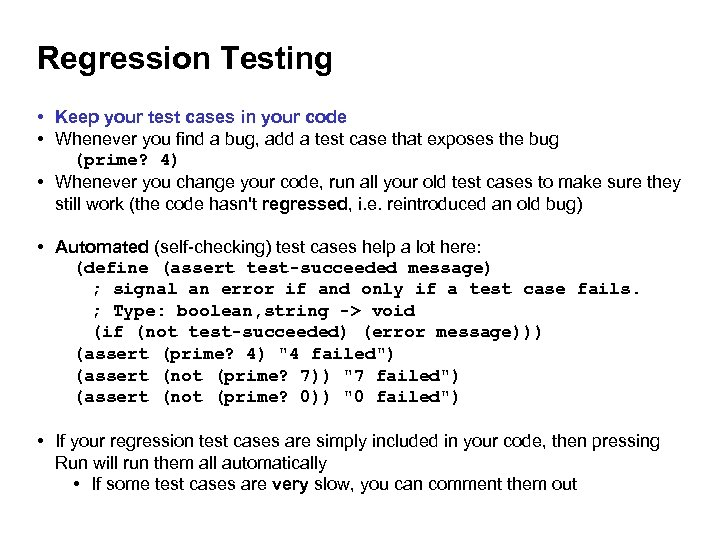Regression Testing • Keep your test cases in your code • Whenever you find a bug, add a test case that exposes the bug (prime? 4) • Whenever you change your code, run all your old test cases to make sure they still work (the code hasn't regressed, i. e. reintroduced an old bug) • Automated (self-checking) test cases help a lot here: (define (assert test-succeeded message) ; signal an error if and only if a test case fails. ; Type: boolean, string -> void (if (not test-succeeded) (error message))) (assert (prime? 4) "4 failed") (assert (not (prime? 7)) "7 failed") (assert (not (prime? 0)) "0 failed") • If your regression test cases are simply included in your code, then pressing Run will run them all automatically • If some test cases are very slow, you can comment them out